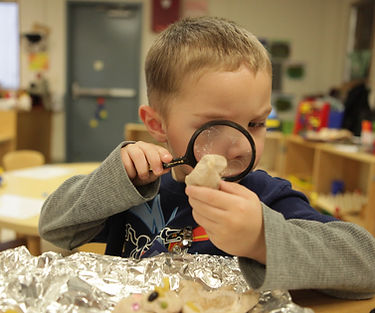Back to Learning Progressions

### Learning Progression:

Students have experience telling and writing time from analog and digital clocks to the hour and half hour in first grade and in five-minute intervals in second grade.

In grade three, students write time to the nearest minute and measure time intervals in minutes. Students solve word problems involving addition and subtraction of time intervals in minutes and represent these problems on a number line (3.MD.1 ).

Students begin to understand the concept of continuous measurement quantities, and they add, subtract, multiply, or divide to solve one-step word problems involving such quantities. Multiple opportunities to weigh classroom objects and fill containers will help students develop a basic understanding of the size and weight of a liter, a gram, and a kilogram.